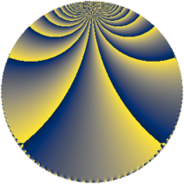# Properties

 Label 648.4.lLevel $648$ Weight $4$ Character orbit 648.l Rep. character $\chi_{648}(107,\cdot)$ Character field $\Q(\zeta_{6})$ Dimension $284$ Sturm bound $432$

# Related objects

## Defining parameters

 Level: $$N$$ $$=$$ $$648 = 2^{3} \cdot 3^{4}$$ Weight: $$k$$ $$=$$ $$4$$ Character orbit: $$[\chi]$$ $$=$$ 648.l (of order $$6$$ and degree $$2$$) Character conductor: $$\operatorname{cond}(\chi)$$ $$=$$ $$72$$ Character field: $$\Q(\zeta_{6})$$ Sturm bound: $$432$$

## Dimensions

The following table gives the dimensions of various subspaces of $$M_{4}(648, [\chi])$$.

Total New Old
Modular forms 672 292 380
Cusp forms 624 284 340
Eisenstein series 48 8 40

## Trace form

 $$284q + 2q^{4} + O(q^{10})$$ $$284q + 2q^{4} - 36q^{10} + 2q^{16} - 8q^{19} - 14q^{22} - 3346q^{25} + 252q^{28} - 1004q^{34} - 198q^{40} + 4q^{43} - 216q^{46} + 6374q^{49} - 1044q^{52} + 612q^{58} + 3956q^{64} + 4q^{67} - 450q^{70} - 8q^{73} + 2920q^{76} - 1376q^{82} - 6242q^{88} + 2736q^{91} + 2808q^{94} + 4q^{97} + O(q^{100})$$

## Decomposition of $$S_{4}^{\mathrm{new}}(648, [\chi])$$ into newform subspaces

The newforms in this space have not yet been added to the LMFDB.

## Decomposition of $$S_{4}^{\mathrm{old}}(648, [\chi])$$ into lower level spaces

$$S_{4}^{\mathrm{old}}(648, [\chi]) \cong$$ $$S_{4}^{\mathrm{new}}(72, [\chi])$$$$^{\oplus 3}$$$$\oplus$$$$S_{4}^{\mathrm{new}}(216, [\chi])$$$$^{\oplus 2}$$